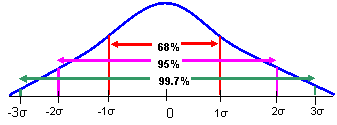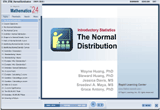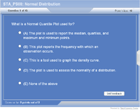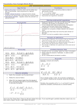How to Learn in 24 Hours?The Rapid Learning Movie

 Need Help? M-F: 9am-5pm(PST): Toll-Free: (877) RAPID-10 US Direct: (714) 692-2900 Int'l: 001-714-692-2900 24/7 Online Technical Support: The Rapid Support Center Secure Online Order:Got Questions? Frequently Asked Questions
 Need Proof? Testimonials by Our Users
 Trustlink is a Better Business Bureau Program. Rapid Learning Center is a fivr-star business. External TrustLink Reviews

 Rapid Learning Courses: MCAT in 24 Hours (2015-16) USMLE in 24 Hours (Boards) Chemistry in 24 Hours Biology in 24 Hours Physics in 24 Hours Mathematics in 24 Hours Psychology in 24 Hours SAT in 24 Hours ACT in 24 Hours AP in 24 Hours CLEP in 24 Hours DAT in 24 Hours (Dental) OAT in 24 Hours (Optometry) PCAT in 24 Hours (Pharmacy) Nursing Entrance Exams Certification in 24 Hours eBook - Survival Kits Audiobooks (MP3)

 Tell-A-Friend: Have friends taking science and math courses too? Tell them about our rapid learning system.Home »  Mathematics »  Introductory Statistics

The Normal Distribution

 Topic Review on "Title": Normal Distribution: describes a set of observations whose graph is symmetric and unimodal. Normal Curve: is a special density curve that characterizes the normal distribution. Parameters: numbers that help specify the model.  For the normal distribution, the parameters are µ for the mean and σ for the standard deviation, written as N(µ, σ) . Z-Score: standardized value of an observation that measures its distance from the mean. 68-95-99.7 Rule:  A sometimes termed the empirical rule, states that, in the Normal model: 68% of the observations in a distribution fall within 1 standard deviation of the mean, 95% of the observations in the distribution fall within 2 standard deviations of the mean, and 99.7% of the observations in the distribution fall within three standard deviations of the mean. Normal quantile plot: A graph used to verify the normality of a distribution. Standardize observations in order to compare values with different units. z-score = (x - μ)/ σ The z-score has no units.  The z-score is an indication of how unusual a value is by how far away from the mean it is. Standardizing shifts the mean to zero and standard deviation to 1.  Standardizing data does not change the shape of the graph. When working problems involving z-score and the 68-95-99.7 rule…draw a picture! x = any given observation σ =standard deviation μ = mean 68-95-99.7 Rule Used for finding the areas within a normal distribution.Rapid Study Kit for "Title":
 Flash Movie Flash Game Flash Card Core Concept Tutorial Problem Solving Drill Review Cheat Sheet"Title" Tutorial Summary : This tutorial detailing normal distribution – describes a set of observations whose graph is symmetric and unimodal. Many important concepts such as why and how data values are standardized, how to use z-scores to calculate area under the normal curve, how to use the 68-95-99.7 Rule, how to calculate z-score based on given information and to identify normal distributions by their normal quantile plot are all clearly demonstrated.

 Tutorial Features: Specific Tutorial Features: Step by step examples showing 68-95-99.7 rule is shown in this tutorial. Examples displaying how to find the z-score are presented with the use of graphs. Series Features: Concept map showing inter-connections of new concepts in this tutorial and those previously introduced. Definition slides introduce terms as they are needed. Visual representation of concepts Animated examples—worked out step by step A concise summary is given at the conclusion of the tutorial.

 "Title" Topic List: Normal Distribution Normal Curve Parameters Z-Score 68-95-99.7 Rule Normal quantile plot z-score Standardizing

See all 24 lessons in Introductory Statistics, including concept tutorials, problem drills and cheat sheets:
Teach Yourself Introductory Statistics Visually in 24 Hours